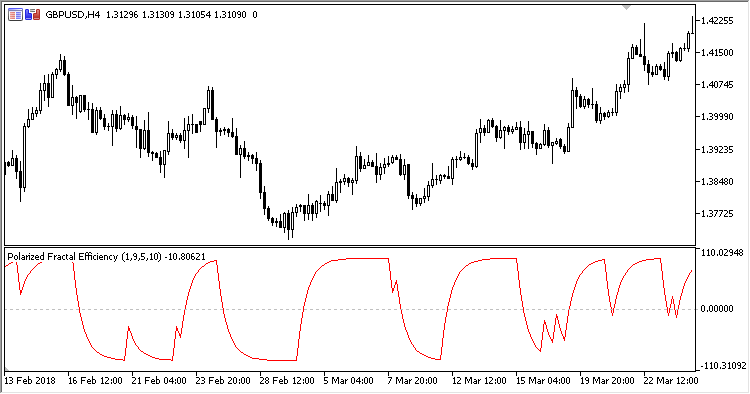793

2018.10.02 09:16

• Fast ROC period - 快速 ROC（变化率）计算周期;
• Slow ROC period - 慢速 ROC（变化率）计算周期;
• MA period - 均线计算周期;
• PDS - 振荡器比例因子。

`PFE = EMA(B, MA period)`

• 如果 Close > Close[Slow ROC period]:

`B = 100.0 * Z`
• 否则:

`B = -100 * Z`
```Z = Sqrt(LongROC * LongROC + 100) / (Sqrt(ShortROC * ShortROC+1) + PDS)
LongROC = 100.0 * (Close / Close[Slow ROC period]-1)
ShortROC = 100.0 * (Close / Close[Fast ROC period]-1)
```

• 振荡器数值高于零 - 上行;
• 振荡器数值低于零 - 下行。智能系统 IchimokuExp_XWAMI_NN3_MMRecPFE_OverlayROCX

ROCX 是一款改编后的 ROC（变化率）指标，显示绝对或相对价格变化。